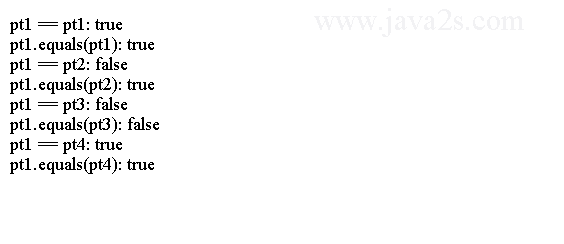Java Object Oriented Design - Java Object Equals

The following code shows how to implement equals() and hashCode() Methods

```class Point {/*  w w  w  .j a  v  a2 s  . c o m*/
private int x;
private int y;

public Point(int x, int y) {
this.x = x;
this.y = y;
}

/* implement the equals() method */
public boolean equals(Object otherObject) {
// Are the same?
if (this == otherObject) {
return true;
}

// Is otherObject a null reference?
if (otherObject == null) {
return false;
}

// Do they belong to the same class?
if (this.getClass() != otherObject.getClass()) {
return false;
}

// Get the reference of otherObject in a SmartPoint variable
Point otherPoint = (Point) otherObject;

// Do they have the same x and y co-ordinates
boolean isSamePoint = (this.x == otherPoint.x && this.y == otherPoint.y);

return isSamePoint;
}

/*
* implement hashCode() method of the Object class, which is a requirement
* when you implement equals() method
*/
public int hashCode() {
return (this.x + this.y);
}
}

public class Main {
public static void main(String[] args) {
Point pt1 = new Point(10, 10);
Point pt2 = new Point(10, 10);
Point pt3 = new Point(12, 19);
Point pt4 = pt1;

System.out.println("pt1 == pt1: " + (pt1 == pt1));
System.out.println("pt1.equals(pt1): " + pt1.equals(pt1));

System.out.println("pt1 == pt2: " + (pt1 == pt2));
System.out.println("pt1.equals(pt2): " + pt1.equals(pt2));

System.out.println("pt1 == pt3: " + (pt1 == pt3));
System.out.println("pt1.equals(pt3): " + pt1.equals(pt3));

System.out.println("pt1 == pt4: " + (pt1 == pt4));
System.out.println("pt1.equals(pt4): " + pt1.equals(pt4));
}
}
```

The code above generates the following result.Note

Here are specifications for the equals() method's implementation. Assume that x, y, and z are non-null references of three objects.

• Reflexivity. The expression x.equals(x) should return true.
• Symmetry. If x.equals(y) returns true, y.equals(x) must return true.
• Transitivity. If x.equals(y) returns true and y.equals(z) returns true, x.equals(z) must return true.
• Consistency. If x.equals(y) returns true, it should keep returning true until the state of x or y is modified. If x.equals(y) returns false, it should keep returning false until the state of x or y is modified.
• Comparison with null reference: An object of any class should not be equal to a null reference. The expression x.equals(null) should always return false.
• Relationship with hashCode() method: If x.equals(y) returns true, x.hashCode() must return the same value as y.hashCode().